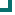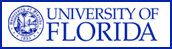Escherichia coli (SOUTHCENTRAL Data) 2004 2005 2006 2007 2008 2009 2010 amoxicillin/clavulanate 91.9%n=234 ampicillin 55%n=1867 51%n=261 ampicillin/sulbactam 61.4%n=1867 52.1%n=261 cefazolin 91.5%n=1867 92%n=261 cefotetan 100%n=275 cefuroxime 92%n=1201 cefotaxime 99%n=509 ceftazidime 97.9%n=1201 96.9%n=261 ceftriaxone 97.6%n=1589 98.1%n=261 cefepime 99.8%n=1867 98.1%n=261 ciprofloxacin 84.4%n=1867 75.9%n=261 levofloxacin 84.7%n=1867 75.9%n=261 gatifloxacin 80.6%n=320 TMP/SMX 76.5%n=814 73.2%n=261 nitrofurantoin 97.9%n=234 tetracycline 73.9%n=234 gentamicin 93%n=1867 82%n=261 tobramycin 94.3%n=1867 85.8%n=261 amikacin 99.1%n=234 aztreonam 97%n=234 imipenem 100%n=1867 100%n=261 piperacillin 65%n=1287 pip/taz 97.1%n=1867 98.1%n=261 ticarcillin/clavulanate 87.9%n=621 Totals 86.9%n=27657 83%n=3393

This data is accurate as of: 11/22/2016 11:54:07 AM

Drugs with less than 10 isolates are removed from the chart and from the graph. The following drugs had some data removed: .ARMProgram.com is a project run by the University of Florida. Copyright 2011. All Rights Reserved. Site by JMarc Technologies, LLC